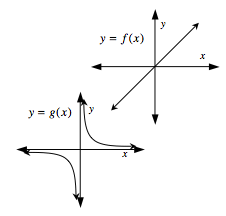### Home > PC > Chapter 6 > Lesson 6.2.3 > Problem6-101

6-101.
1. Copy the graphs of y = f(x) and y = g(x) on the same set of axes. Homework Help ✎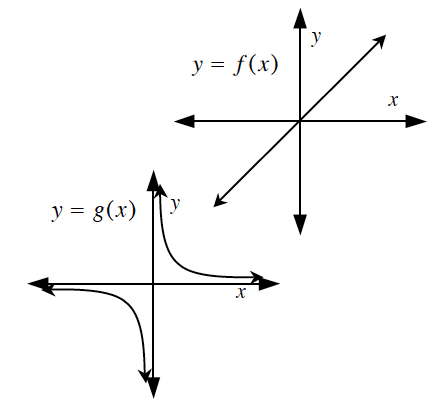1. Use graphical addition to sketch the graph of h(x) = f(x) + g (x).

2. Make a reasonable guess for the equations f(x) and g(x). Use this to test your graph of h(x).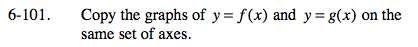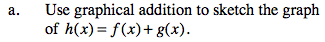Make possible tables for each graph.
Add the y-values to obtain h(x).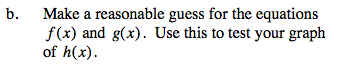f(x) looks like y = x.

$g(x) \text{ looks like }y= \frac{1}{x}.$